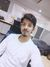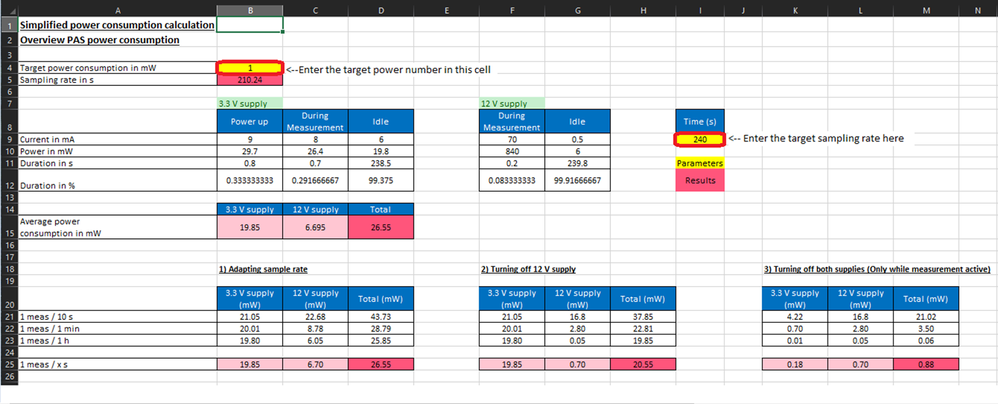# Estimating the power consumption with the XENSIV_PAS_CO2_Power_calculator - KBA234210Community ManagerCommunity Manager# Estimating the power consumption with the XENSIV_PAS_CO2_Power_calculator - KBA234210

Version: **

Community Translation: XENSIV_PAS_CO2_Power_calculatorを使用した消費電力の見積もり - KBA234210

The XENSIV_PAS_CO2_Power_calculator helps you quickly estimate the power consumption and arrive at the power budget when developing a product using the XENSIV™ PAS CO2 sensor.

Enter the Target power consumption in cell B4 of the Overview tab. This automatically list the sampling rate required to meet the target power in cell B5 of the Overview tab. For example, if the desired target power is 1 mW, the sampling rate is calculated as 210.24 s.

You can also do the converse calculation (power consumption for a given sampling rate). To do this, enter the sampling rate in cell I9 of the Overview tab. You get detailed estimates of the following parameters:

1. Idle time in seconds when using both the 3.3-V and 12-V supply, and its duration as a percentage of the power-up measurement and idling.
2. Average power consumption of 3.3 V and 12 V along with the total average power consumption.
3. Average power consumed when the 12-V power supply is turned off, and when both the 3.3-V and the 12-V supplies are turned off.

The Excel sheet also provides standard power numbers when the measurement frequency is every 10 seconds, 1 minute, and 1 hour (items 2 and 3 in the list above).

See Figure 1 for an example. See the Help tab of the XENSIV_PAS_CO2_Power_calculator spreadsheet for more information.Figure 1

551 Views